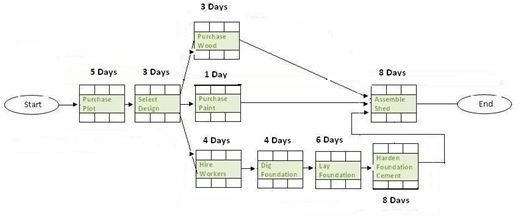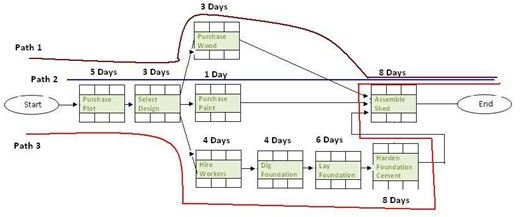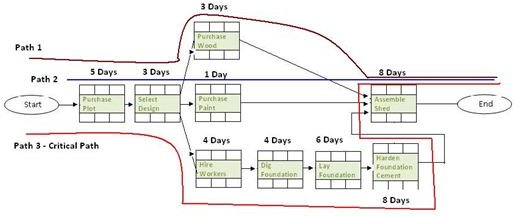# Critical Path Example - Identify the Critical Path in a Precedence Diagram

Page content

## Critical Path Example Using A Precedence Diagram

A project usually consists of multiple activities that occur both simultaneously and sequentially. To determine the flow of these activities, you’ll need to create a Precedence Diagram. After creating the Precedence Diagram, you can identify the activities that would, if delayed, cause your project to come in late. This is the Critical Path definition. A delay in any of the critical path activities will delay the entire project, regardless of whether the other project activities are completed on or before time. This article will walk you through a critical path example using a precedence diagram.Critical path example from Wikimedia Commons The act of determining the Critical Path is known as the Critical Path Method or the Critical Path Analysis. To learn about the pros and cons of the critical path method, read the Advantages and Disadvantages of the Critical Path Method article. To determine the Critical Path and conduct Critical Path Analysis, you need to:

1. Define the duration of each activity.
2. Identify all the paths.
3. Calculate the duration of each path.
4. Identify the longest path.

Let’s consider some critical path examples.

## Step 1: Define the Duration of Each Activity

You are managing the construction of a shed. You have created a Precedence Diagram and defined the estimated duration of each activity, as shown in the image below. (Click any image in this article for a larger view.)Precedence Diagram Activity Duration

For more about estimation best practices, read the Realistic Project Estimation Techniques article. The article will help you determine accurate activity duration and therefore enable you to use this method or analysis accurately.

## Step 2: Identify All the Paths

The Precedence Diagram shown in the image has three paths, as shown below:Calculating Precedence Diagram Paths

One of the paths in the image above is the Critical Path. By using the Critical Path Method or the Critical Path Analysis we can determine the critical path. Can you read the critical path definition and guess the critical path?

## Step 3: Calculate the Duration of Each Path

To do this, you can simply add the duration of each activity in each path. For Path 1, you get:

Duration for Path 1 = Duration of Start + Duration of Purchase Plot + Duration of Select Design + Duration of Purchase Wood + Duration of Assemble Shed + Duration of End

Now, plug in the numbers and you get:

Duration of Path 1 = 0 days + 5 days + 3 days + 3 days + 8 days + 0 days = 19 days

Note: The duration of Start and End is zero because there aren’t activities, rather they are events.

Similarly, you can calculate the duration of the other paths:

Duration of Path 2 = 0 days + 5 days + 3 days + 1 days + 8 days + 0 days = 17 days

Duration of Path 3 = 0 days + 5 days + 3 days + 4 days + 4 days + 6 days + 8 days + 8 days + 0 days = 38 days

The duration calculated above assume that there is no scope creep. In real projects, scope creep can be expected. However, it is up to the project manager to control the degree of scope creep. Read the Managing Scope Creep article for more about controlling and monitoring scope creep. Each path has a different duration. That means the Critical Path Method or the Critical Path Analysis is required to determine the most important path. As a project manager, you will need to keep an eye on the Critical Path.

## Step 4: Identify the Longest Path

The longest path is the critical path. From the above calculations, we can see that Path 3 is the longest. Therefore, if activity on this path is delayed, then the project will be delayed. After identifying the critical path, you can deduce the activities that when delayed will not impact the project.Precedence Diagram Critical Path

For example, do you think the project will be delayed if the “Purchase Wood” activity is delayed by five days? The answer is no, the project will not be delayed. What if the “Purchase Wood” activity came in 8 days late? Read Calculate the Float of Each Activity Using the Critical Path Method (CPM) to determine the amount of time an activity may be late without delaying the project. As you can see, the critical path method or critical path analysis is very useful for determining activities that can lead to project delay. Critical path examples can get complex, but in theory the critical path method and critical path definition is always the same and follows the steps mentioned in this article. Last, you might want to also read Risk Management practices that can help you protect your project from time and cost over-runs.